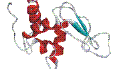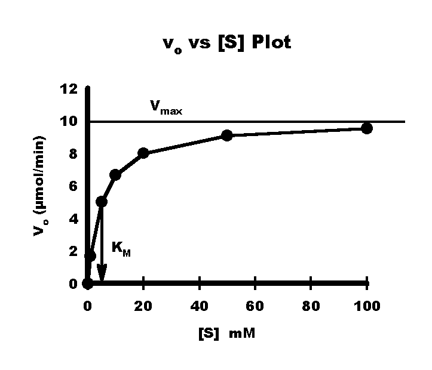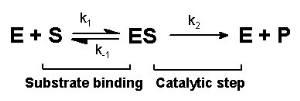Home   |    Site Map   |    Copyright   |    Contact us   |    Privacy   |Enzymes
Enzyme classes:
EC 1: Oxidoreductases
EC 2: Transferases
EC 3: Hydrolases
EC 4: Lyases
EC 5: Isomerases
EC 6: Ligases
General information:
Catalytic mechanism
Enzyme kinetics
Inhibitors
Enzymes in industry

# Enzyme kinetics

## General principles of enzyme kinetics

Enzymes acts as a catalysts. Enzymes do not change chemical equilibrium of reaction, this is very important point to remember. The only way to utilize all substrate is to remove products after reaction. The enzymatic catalysis can be shown by following equations:
E + S <=> ES <=> E + P

Where E is enzyme, S - substrate, ES - enzyme-substrate complex, P - product. The second part of reaction can be consider as irreversible at the beginning of reaction, when the concentration of product is significantly lower than substrate concentration.

In many reactions in vivo and in vitro concentration of enzyme is much lower than concentration of substrate. In this case all enzyme molecules are involved into catalysis, or they will become saturated. In these conditions enzyme will react with maximum velocity (Vmax). This value is easily observe in experiment and this will allow to calculate parameters of enzyme kinetics.

## Michaelis-Menton kinetics equation

Because all reactions catalysed by enzymes are saturable, the dependence of reaction rate over the substrate concentration is not linear:Michaelis-Menten saturation curve - Vo vs. [S] plotSingle substrate reaction. k1, k-1 and k2 are constants for individual reactions.
Taking into account that concentration of product is low, we can use this equation to describe enzymatic catalysis. The final velocity is v=k2[ES]. At very high concentration of substrate, all enzyme is saturated and v~k2[E]tot~Vmax, where [E]tot = [E]+[ES] it is possible to derive Michaelis-Menten equation:

v=(Vmax[S])/(Km + [S])

where Km=(k2+k-1)/k1 ~ ([E][S])/[ES] - Michaelis constant.

The classical situation (Briggs-Haldane kinetics), when k2 is << k-1 at the initial period the reaction velocity is constant it is possible to derive another form of Michaelis-Menten equation:

v=k2[ES]=k2[E][S]/Km